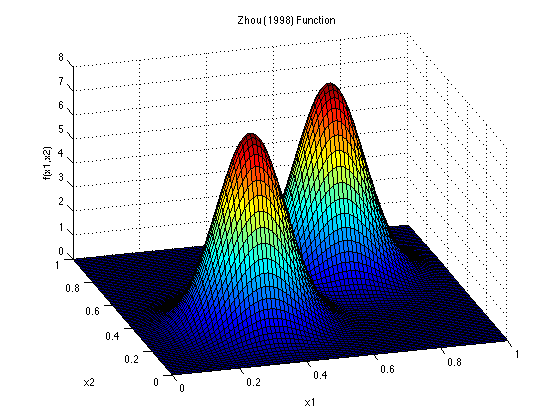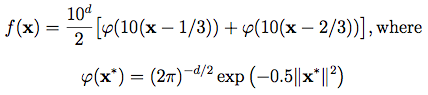Zhou (1998) FunctionDescription:

Dimensions: d

This function is considered by Zhou (1998) for the numerical integration of spiky functions. An & Owen (2001) approximate the function in d = 9 dimensions. As they point out, this function is such that there is no reason to expect it to be approximately a low-order polynomial.

In the equations above, ‖·‖ is the Euclidean norm of a vector, and the operations 10(x-1/3) and 10(x-2/3) are completed component-wise on the vector x.

Input Domain:

For the purposes of emulation/prediction, the function is evaluated on xi ∈ [0, 1], for all i = 1, …, d.

For integration purposes, when the function is integrated over [0, 1]d, the integral is slightly smaller than 1.

References:

An, J., & Owen, A. (2001). Quasi-regression. Journal of Complexity, 17(4), 588-607.

Zhou, Y. (1998). Adaptive importance sampling for integration. Ph.D. Thesis, Stanford University.